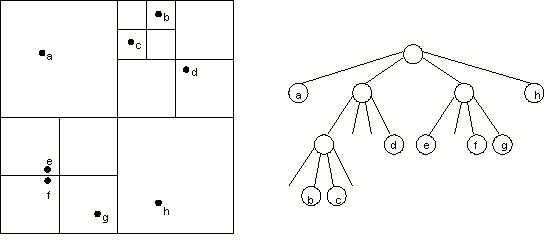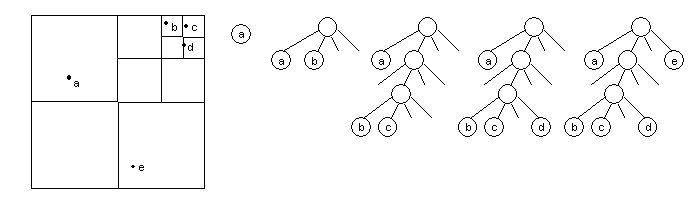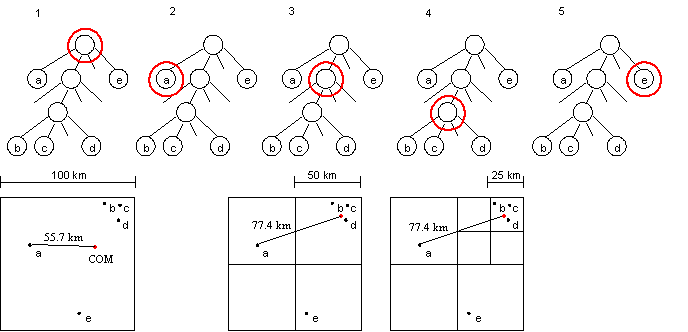COS 126 N-Body Simulation Programming Assignment

In the N-body assignment, you created a brute force program to simulate the motion of N bodies, mutually affected by gravitational forces. In this brute force solution, the number of force calculations per step is proportional to N2. The brute force algorithm is not practical when N is large. In this assignment, you will implement the Barnes-Hut algorithm to simulate each step in time proportional to N log N and animate the evolution of entire galaxies. The Barnes-Hut algorithm is the algorithm most scientists use for N-Body simulation.

The Barnes-Hut algorithm. The crucial idea in speeding up the brute force algorithm is to group nearby bodies and approximate them as a single body. If the group is sufficiently far away, we can approximate its gravitational effects by using its center of mass. The center of mass of a group of bodies is the average position of a body in that group, weighted by mass. Formally, if two bodies have positions (x1 , y1) and (x2, y2), and masses m1 and m2, then their total mass and center of mass (x, y) are given by:

m = m1 + m2
x = (x1m1 + x2m2) / m
y = (y1m1 + y2m2) / m
The Barnes-Hut algorithm is a clever scheme for grouping together bodies that are sufficiently nearby. It recursively divides the set of bodies into groups by storing them in a quad-tree. A quad-tree is similar to a binary tree, except that each node has 4 children (some of which may be empty). Each node represents a region of the two dimensional space. The topmost node represents the whole space, and its four children represent the four quadrants of the space. As shown in the diagram, the space is recursively subdivided into quadrants until each subdivision contains 0 or 1 bodies (some regions do not have bodies in all of their quadrants. Hence, some internal nodes have less than 4 non-empty children). Each external node represents a single body. Each internal node represents the group of bodies beneath it, and stores the center-of-mass and the total mass of all its children bodies. Here is an example with 8 bodies.To calculate the net force on a particular body, traverse the nodes of the tree, starting from the root. If the center-of-mass of an internal node is sufficiently far from the body, approximate the bodies contained in that part of the tree as a single body, whose position is the group's center of mass and whose mass is the group's total mass. The algorithm is fast because we don't need to individually examine any of the bodies in the group. If the internal node is not sufficiently far from the body, recursively traverse each of its subtrees. To determine if a node is sufficiently far away, compute the quotient s / d, where s is the width of the region represented by the internal node, and d is the distance between the body and the node's center-of-mass. Then, compare this ratio against a threshold value θ. If s / d < θ, then the internal node is sufficiently far away. By adjusting the θ parameter, we can balance the speed and accuracy of the simulation. We will always use θ = 0.5, a value commonly used in practice. Note that if θ = 0, then no internal node is treated as a single body, and the algorithm degenerates to brute force.

Constructing the Barnes-Hut tree. To construct the Barnes-Hut tree, insert the bodies one after another. To insert a body b into the tree rooted at node x, use the following recursive procedure:

1. If node x does not contain a body, put the new body b here.
2. If node x is an internal node, update the center-of-mass and total mass of x. Recursively insert the body b in the appropriate quadrant.
3. If node x is an external node, say containing a body named c, then you now have bodies b and c in the same region. Subdivide the region further by creating four children. Then, recursively insert both b and c into the appropriate quadrant(s). Since b and c may still end up in the same quadrant, there may be several subdivisions during a single insertion. Finally, update the center-of-mass and total mass of x.

As an example, consider the 5 bodies in the diagram below. In our examples, we use the convention that the branches, from left to right, represent the northwest, northeast, southwest, and southeast quadrants, respectively. The tree goes through the following stages as the bodies are inserted:The root node contains the center-of-mass and total mass of all five bodies. The two other internal nodes each contain the center-of-mass and total mass of the bodies b, c, and d.

Calculating the force acting on a body. To calculate the net force acting on body b, use the following recursive procedure, starting with the root of the quad-tree:

1. If the current node is an external node (and it is not body b), calculate the force exerted by the current node on b, and add this amount to b's net force.
2. Otherwise, calculate the ratio s / d. If s / d < θ, treat this internal node as a single body, and calculate the force it exerts on body b, and add this amount to b's net force.
3. Otherwise, run the procedure recursively on each of the current node's children.
As an example, to calculate the net force acting on body a, we start at the root node, which is an internal node. It represents the center-of-mass of the five bodies a, b, c, d, and e, which have masses 1, 2, 3, 4, and 5 kg, respectively. The force calculation proceeds as follows:1. The first node examined is the root. The quotient s/d = 100/55.7 > θ = 0.5, so we perform the process recursively on each of the root's children.
2. The first child is body a itself. A node does not exert force on itself, so we don't do anything.
3. This child represents the northeast quadrant of the space, and contains the center-of-mass of bodies b, c, and d. Now s/d = 50/77.4 > θ so we recursively calculate the force exerted by the node's only child.
4. This is also an internal node, representing the northeast quadrant of its parent, and containing the center-of-mass of bodies b, c, and d. Now s/d = 25/77.4 < θ. Treating the internal node as a single body whose mass is the sum of the masses of b, c, and d, we calculate the force exerted on body a, and add this value to the net force exerted on a. Since the parent of this node has no more children, we continue examining the other children of the root.
5. The next child is the one containing body e. This is an external node, so we calculate the pairwise force between a and e, and add this to a's net force.

Your Assignment. Implement the Barnes-Hut algorithm and animate the results using StdDraw.

• Brute force implementation. In Assignment 2, you implemented a brute force solution. We provide an object-oriented version of that assignment that uses an array of Body objects. Because we are now interested in plotting thousands of bodies, we plot each one as a single pixel instead of with an image. Accordingly, the input format replaces the name of the picture file with three integers (r, g, b) that encode the RGB color. Otherwise the format is identical to Assignment 2. Download Body.java and NBodyBrute.java. Test the code on a few of the smaller data sets. As you can see, the program becomes sluggish very quickly as the size of the input file grows.

• Create a data type for quadrants. In the Barnes-Hut algorithm, given a body, you must determine in which subdivision of the plane it lies. Create a data type Quad to represent a square subdivision of the plane. Implement the following public interface:
• `public Quad(double xmid, double ymid, double length)`: create a new quadrant centered at (xmid, ymid) of the given side length.
• `public boolean contains(double x, double y)`: Return true if (x, y) is in the quadrant, and false otherwise.
• `public double length()`: Returns the length of a side of the quadrant.
• `public Quad NW(), NE(), SW(), and SE()`: These four methods create and return a new `Quad` representing a sub-quadrant of the invoking quadrant.

• public String toString(): return string representation of the quad.

• public void draw(): draw the quad using StdDraw.

• Modify the Body data type. The Body data type now represents both individual bodies (external nodes of the Barnes-Hut tree) and groups of bodies (internal nodes). For an internal node, the data member mass represents the total mass of a group of bodies, rx represents the x-coordinate of the group's center of mass, and ry represents the y-coordinate. The remaining data members can be ignored. Add the following methods to the Body data type.
• `public boolean in(Quad q)`: Returns true if the invoking body is in quadrant `q` and false otherwise.
• `public Body plus(Body b)`: return a new Body that represents the center-of-mass of the the invoking body and b, using the center-of-mass formula provided above.

• Create the Barnes-Hut tree data type. This is the trickiest part of the assignment. A Barnes-Hut tree contains some data (a body and a quadrant), plus four references to other Barnes-Hut trees. In Java, we can define it as below.
```public class BHTree {
private Body body;     // body or aggregate body stored in this node
private BHTree NW;     // tree representing northwest quadrant
private BHTree NE;     // tree representing northeast quadrant
private BHTree SW;     // tree representing southwest quadrant
private BHTree SE;     // tree representing southeast quadrant
}
```
You must implement the following constructors and methods:

• public BHTree(Quad q): create a Barnes-Hut tree with no bodies, representing the given quadrant.

• public void insert(Body b): add the body b to the invoking Barnes-Hut tree.

• public void updateForce(Body b): approximate the net force acting on body b from all the bodies in the invoking Barnes-Hut tree, and update b's force accordingly.

• public String toString(): return string representation of the Barnes Hut tree.

• public void draw(): draw the Barnes-Hut tree using StdDraw.

• Implement the Barnes-Hut algorithm. Create a program NBody.java, based on NBodyBrute.java, that performs the Barnes-Hut algorithm. For each time step of the simulation (use dt = .1), create a new Barnes-Hut tree from scratch, and insert all of the bodies. Since the Barnes-Hut tree represents a finite region in the plane, only insert those bodies that are inside the universe. After inserting all of the bodies, reset the net force acting on each body and call `updateForce()` to recalculate it. Then, update the positions of the bodies and plot them.
This assignment was developed by Tom Ventimiglia and Kevin Wayne.